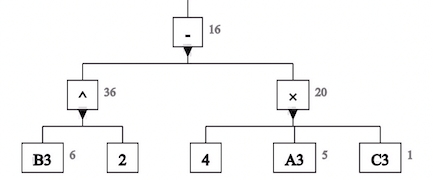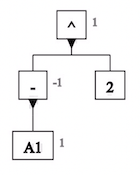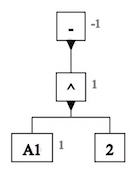# The Tree View

Formulas in Excel specify computations to perform on values represented by constants and on values it finds in spreadsheet cells. The tree view portrays a formula in a way that clarifies the order in which these computations are performed.

For example, the tree view for the discriminant in the quadratic formula shows that Excel evaluates the power `B2^2` and the product `4*A3*C3` before it evaluates their difference. The lowest rectangles in the diagram contain labels for primitive values: constants (`2` and `4`) and references (`A3`, `B3`, and `C3`) to values in spreadsheet cells. Above them are rectangles that contain operators: exponentiation `^`, multiplication `×`, and subtraction `-`. Next to each rectangle for a reference or operator is its value.Tree view for B3^2 - 4*A3*C3

The tree view is particularly valuable when formulas are long and complicated, or when Excel does not evaluate them in the order one might expect. For example, the tree view shows that Excel evaluates `-A1^2` as if it had been written `(-A1)^2` and that one must write `-(A1^2)` to get the operations performed in the other order.-A1^2(-A1)^2-(A1^2)

## Structure of the tree view

The name tree view is motivated by that fact that such diagrams look like (upside down) trees. Each rectangle in the tree view represents an element in a formula and contains a symbol that identifies the type of that element:

• a constant (e.g., `2` or `"New York"`),
• a reference to a cell or range (e.g., `A1` or `B1:B2`),
• a user-defined name (e.g., `price` or `state`),
• an operator (e.g., `+` or `*`), or
• a function (e.g., `SQRT` or `IF`).

For increased readability, the tree view substitutes common mathematical symbols for the notations used by Excel:

• `×` for `*`,
• `≤` for `<=`,
• `≥` for `>=`,
• `≠` for `<>`,
• `∪` for a comma used as a range union operator,
• `∩` for a space used as a range intersection operator,
• `{...}` for an array constant, and
• `...` for a row in an array constant.

Each rectangle that contains an operator or a function represents a decomposable expression. Immediately beneath it in the tree view are other rectangles, called its children and connected to it as in an organization chart or a family tree, that represent the operator's operands or the function's arguments. Thus elements representing `4`, `A3`, and `C3` are children of an element representing `4*A3*C3`, and an element representing `A1` is a child of an element representing `SQRT(A1)`.

The lowest elements in the tree view represent indecomposable elements: constants, references, user-defined names, and functions without any arguments (e.g., `PI()`).

No parentheses appear in the tree view.